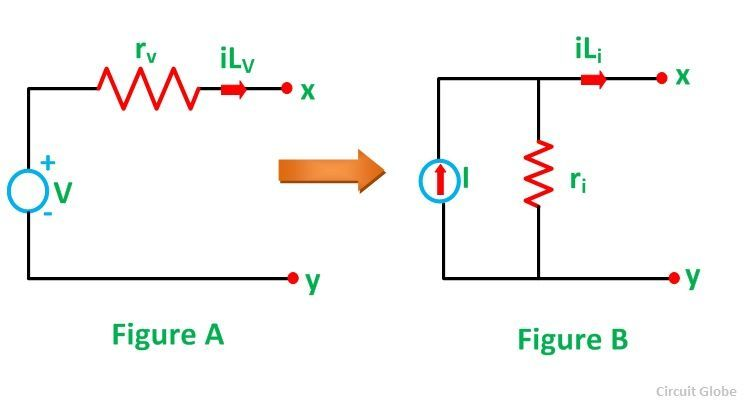# Source Transformation

Source Transformation simply means replacing one source by an equivalent source. A practical voltage source can be transformed into an equivalent practical current source and similarly a practical current source into voltage source.

Any practical voltage source or simply a voltage source consists of an ideal voltage source in series with an internal resistance or impedance (for an ideal source this impedance will be zero), the output voltage becomes independent of the load current. Cells, batteries and generators are the example of the voltage source.

For any practical current source or simply current source, there is an ideal current source in parallel with the internal resistance or impedance, for ideal current source this parallel impedance is infinity.

The semiconductor devices like transistors, etc. are treated as a current source or an output produce by the direct or alternating voltage source is called direct and alternating current source, respectively.

The voltage and current source are mutually transferable or in other words the source transformation i.e. voltage to the current source and current to a voltage source can be done. Let us understand this by considering a circuit given below:Figure A represents a practical voltage source in series with the internal resistance rv, while figure B represents a practical current source with parallel internal resistance ri

For the practical voltage source the load current will be given by the equation:Where,

iLv is the load current for the practical voltage source
V is the voltage
rv is the internal resistance of the voltage source

It is assumed that the load resistance rL is connected at the terminal x-y. Similarly for the practical current source, the load current is given as:Where,

iLi is the load current for the practical current source

I is the current

ri is the internal resistance of the current source

rL is the load resistance connected across the terminal x-y in the figure B

Two sources become identical, when we will equate equation (1) and equation (2)However, for the current source, the terminal voltage at x-y would be Iri, x-y terminal are open. i.e.

V = I x ri

Therefore, for any practical voltage source, if the ideal voltage is V and internal resistance be rv, the voltage source can be replaced by a current source I with the internal resistance in parallel with the current source.

## Source Transformation: Conversion of Voltage Source into Current SourceWhen the voltage source is connected with the resistance in series and it has to be converted into the current source than the resistance is connected in parallel with the current source as shown in the above figure.

Where Is = Vs /R

## Conversion of Current Source into Voltage SourceIn the above circuit diagram a current source which is connected in parallel with the resistance is transformed into a voltage source by placing the resistance in series with the voltage source.

Where, Vs = Is / R